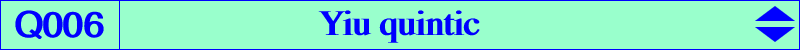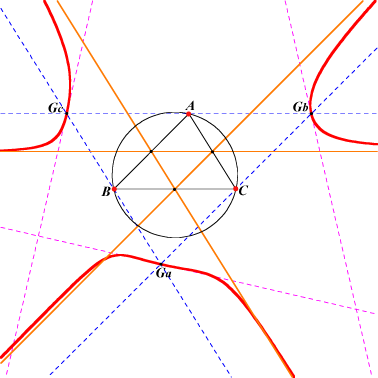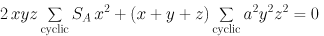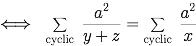A, B, C singular points points at infinity of the sidelines of ABC vertices Ga, Gb, Gc of the antimedial triangle other points belowQ006 is a circular quintic with singular focus X(5). The three real asymptotes are the sidelines of the medial triangle. The tangents at Ga, Gb, Gc are parallel to the sidelines of the tangential triangle. Locus properties See K004 Darboux cubic, property 6. Locus of P such that P is on the tripolar of the cyclocevian conjugate of P (Wilson Stothers, Hyacinthos #13339) Locus of P such that P is on the circumcircle of the cevian triangle of P (Wilson Stothers, Hyacinthos #13345) Let PaPbPc be the cevian triangle of P. The perpendicular bisectors of PPa, PPb, PPc concur if and only if P lies on Q006. These perpendicular bisectors bound a triangle perspective with ABC if and only if P lies on the septic Q096. Let PaPbPc be the cevian triangle of P. The perpendiculars at Pa, Pb, Pc to APa, BPb, CPc concur (at Q) if and only if P lies on Q006 together with the line at infinity (Francisco Garcia Capitan, private message 2016-03-28). The locus of Q is a very complicated 11th degree curve.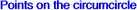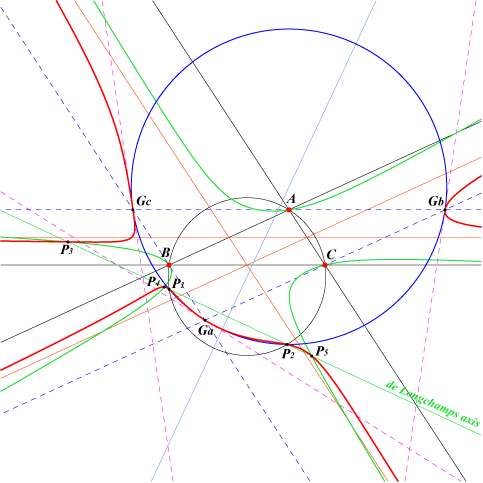When ABC is obtusangle, Q006 meets the de Longchamps axis (trilinear polar of X(76), isotomic transform of the circumcircle) at two real points P1, P2 lying on the circumcircle and also on the circumcircle of GaGbGc. P1 and P2 are clearly isotomic conjugates with midpoint X(858), the projection of X(3) on the de Longchamps axis, also the inverse of X(2) in the nine point circle. The remaining three common points P3, P4, P5 lie on the non-pivotal cubic nK0(X2, Q) where Q is the intersection of the lines X(2)X(39) and X(5)X(83). Q = a^4 + b^4 + c^4 - b^2c^2 : : is now X(7828) in ETC.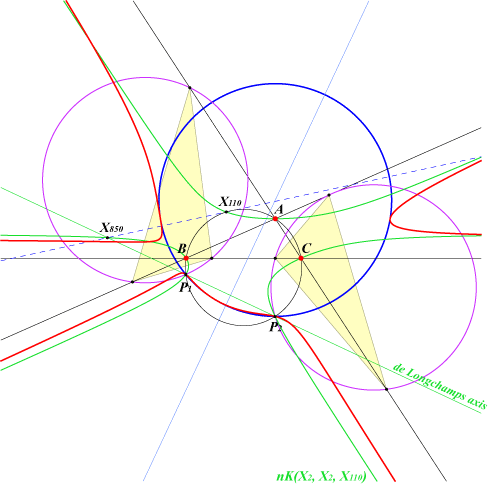The cevian triangles of P1 and P2 are congruent to the cevian triangles of X(110) and X(850). These points lie on the cubic nK(X2, X2, X110), a Tucker cubic. More details on Tucker cubics here.Q006 and the X99-Cevian points Q006 contains the X99-Cevian points. See Table 28 : cevian and anticevian points for explanations.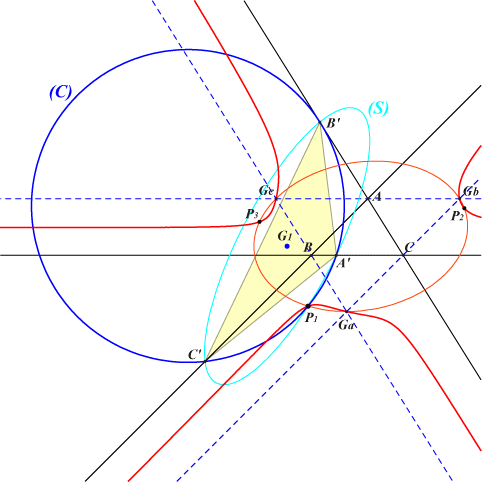There are seven X99-Cevian points Pi although not always all real. These also lie on the Steiner ellipse of the antimedial triangle. If A'B'C' is the cevian triangle of one of these points Pi then Pi is the Steiner point of the triangle A'B'C'. The figure shows a configuration where three of them are real. (C) and (S) are the circumcircle and the Steiner ellipse of the cevian triangle of P1. G1 is the centroid of the triangle.